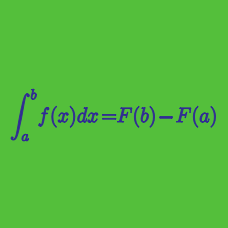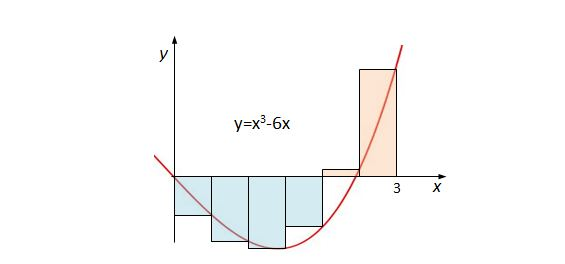Calculus

# Riemann Sums

What is the value of the definite integral $\int_{0}^{1}(9+3x^2)dx?$

Evaluate $\int_{-2}^{-1}\left( x^3+3x^2+7 \right)dx + \int_{-1}^{2}\left( y^3+3y^2+7 \right)dy.$

If $\int_{0}^{10}f(x)dx=25 \text{ and } \int_{0}^{8}2f(x)dx=22,$ what is the value of $\int_{8}^{10}f(x)dx?$Find the Riemann sum for $f(x)=x^3-6x$ using the right endpoints of each subinterval, where $f(x)$ is defined over the interval $[0, 3]$ and the number of subintervals is $n=6.$

Consider the limit $\lim_{n \to \infty} \sum_{i=1}^{n} \left(x_i^{10}+x_i \sin x_i\right) \Delta x.$ Which of the following definite integrals expresses this limit on the interval $[0, \pi]?$

×